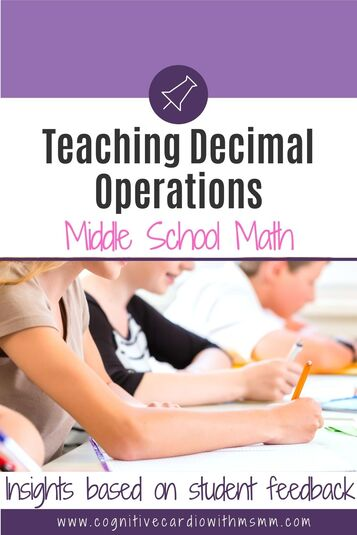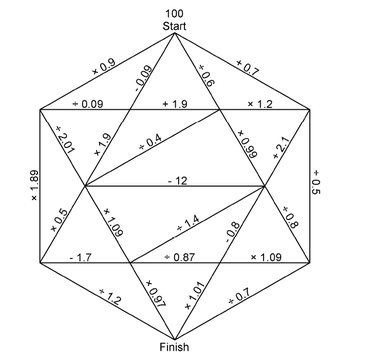# Why I'm Not Teaching Decimal Operations "Rules"

## Teaching Decimal OperationsThere are rules for adding and subtracting decimals. There are rules for multiplying and dividing decimals. How often have you found that your middle school math students have been taught just the math rules, and even some little tricks to remember those rules?

How often do your math students make procedural mistakes even though they can remember the little tricks and they’ve learned the rules?

I’ve taught decimal operations for more than 20 years, and I’ve seen, time and again, students who ‘know’ how to add and multiply decimals but then follow the wrong ‘rule’ for the operation they’re completing.

6th grade math students know there are rules, but they end up asking themselves things like:

• Do I line up decimal points when adding or when multiplying?
• Should I not line up decimal points when multiplying or when subtracting?
• Do I “jump” the decimal point over when adding and subtracting?
• Or do I do that when multiplying?
• What if there’s no decimal point?

They don’t remember when to use which method to place the decimal point.

## Students’ Thinking About Decimal Operations

So, this year, as we approach the decimal unit, I’ve been feeling like I don’t want to talk about the rules for where/how to put the decimal point.
I want to focus on logic.
​Today that feeling was reinforced when I asked my students to solve 35.2 + 7.489 and then explain why their answer made sense. Here are some of the answers and reasons (I didn’t teach this yet, but they learned it last year):

• “0.11009 makes sense because I tried my best and if I remember correctly, addition problems you don’t need to line the decimals together”
• “0.7838 makes sense because when I added I knew that it doesn’t matter how it’s lined up”
• “78.42 – I added 9 and 2, then 1, 8 and 5. Next I added 1, 3, and 7. Finally I added 7 and 0 and I put the decimal in the middle.”
• “7.841 makes sense because with adding you only have to add the decimals on the top. Then you add and finally add the decimal back in.”
• “426.89 because I put the decimal point four spaces back because there are four numbers behind it”
•  “79.41 makes sense because you do it just like an addition problem (that’s how I remember it anyway)”
• “7.841 makes sense because you add like normal and take the decimal from the farthest out and put it with the answer”

A few correct answers, with reasons:

• “42.689 – this makes sense to me because this is how I learned it. You do simple addition, but line up the decimal points”
• “42.689 makes sense because I used what my fifth grade teacher taught me, line up decimals, add zeros so everything is lined up and then solve.”
• “42.689 – I don’t know how it makes sense, but it’s how I learned to do it.”

Of the 120 6th grade students in my math classes, only EIGHT said the answer made sense because:

• “35 + 7 is 42” or because
• “I estimated” or
• “When we’re doing addition, we know we end up with a bigger number.”

​Now, that doesn’t mean that they didn’t think about those things, but to the students, answers seemed to “make sense” when they followed the rules – even if the rules are remembered incorrectly; students got right and wrong answers and they all made sense because that’s “how they learned it.”

## What’s the Point of Teaching Decimal Operation Rules?

So, what is the point of teaching rules?
Especially to those middle school students who are a little weaker in math – if they can’t remember the right rule, they can’t tell if their answer is reasonable! They need to develop their number sense.
In the past, I’ve asked students to estimate the answer first, so they know if their answer is reasonable, and I have required them write these estimates on their tests. But we’ve also talked about the rules.

​I’m thinking that if I take the focus off the rules and put extra focus on the estimating/reasonable answer idea, students will be better able to identify reasonable answers and will feel less dependent on the rules.

## Multiplying and Dividing Decimals

I know the decimal multiplication and division logic will be more difficult.

Problems like 23.5 x 4.428, won’t be as bad because there are whole numbers involved.

• This could be estimated as 25 x 4 = 100.
• So when placing the decimal point in 104058, students should place it so that the answer is about 100…not 10, or 1, or 1000.

​Multiplying 23.5 and 0.7 may be more confusing, but this will be the time to help students understand why the answer should be smaller than 23.5….but more than half of 23, since 0.7 is more than 0.5.I’m sure division will be the most challenging, as far as determining reasonable answers, and I need to think about this one a bit more.

However, we have already done this activity I found on YouCubed -“Too Big or too Small Maze Board.” (I searched for this on the site recently and didn’t see it there any more:-/)

​In attempting to create the largest number possible (using a calculator to compute), many students have already made the discovery that dividing by a number less than one gave them a larger number, while multiplying by a number less than one gave them a smaller number. No rules were taught – they found this “secret” on their own. This will be great to reference and discuss when we begin working on the multiplication and division of decimals.

We’ll see how it goes!

How do you teach decimal operations?

## EllieWelcome to Cognitive Cardio Math! I’m Ellie, a wife, mom, grandma, and dog ‘mom,’ and I’ve spent just about my whole life in school! With nearly 30 years in education, I’ve taught:

• All subject areas in 4th and 5th grades
• Math, ELA, and science in 6th grade (middle school)

I’ve been creating resources for teachers since 2012 and have worked in the elearning industry for about five years as well!